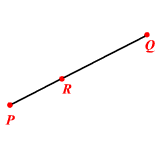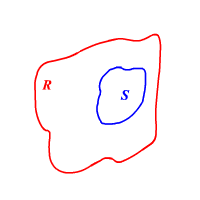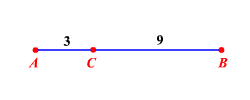# Geometric Probability

The Probability of an event represents the chance that the even will occur. Geometric probability describes the chance that a point lies on a part of a line segment or on a part of a region.

Consider the line segment $\stackrel{¯}{PQ}$ Suppose a point $X$ is picked at random.Then,

probability of $X$ is on $\stackrel{¯}{PR}=\frac{\text{Length}\text{\hspace{0.17em}}\text{\hspace{0.17em}}\text{of}\text{\hspace{0.17em}}\stackrel{¯}{PR}\text{\hspace{0.17em}}}{\text{Length}\text{\hspace{0.17em}}\text{\hspace{0.17em}}\text{of}\text{\hspace{0.17em}}\stackrel{¯}{PQ}}$ .

Consider the region $R$ and the region $S$ . Suppose a point $P$ is picked at random.Then,

probability of $P$ is in $S=\frac{\text{Area}\text{\hspace{0.17em}}\text{\hspace{0.17em}}\text{of}\text{\hspace{0.17em}}\text{\hspace{0.17em}}S}{\text{Area}\text{\hspace{0.17em}}\text{\hspace{0.17em}}\text{of}\text{\hspace{0.17em}}\text{\hspace{0.17em}}R}$ .

Example:

Find the probability that a point chosen at random lies on $\stackrel{¯}{AC}$ .Probability of the point is on $\stackrel{¯}{AC}=\frac{\text{Length}\text{\hspace{0.17em}}\text{\hspace{0.17em}}\text{of}\text{\hspace{0.17em}}\text{\hspace{0.17em}}AC}{\text{Length}\text{\hspace{0.17em}}\text{\hspace{0.17em}}\text{of}\text{\hspace{0.17em}}\text{\hspace{0.17em}}AB}$

Use the Segment Addition Postulate to find the length of $\stackrel{¯}{AB}$

$\begin{array}{l}AB=AC+CB\\ =3+9\\ =12\end{array}$

Substitute.

Probability of the point is on $\stackrel{¯}{AC}=\frac{3}{12}$

$=\frac{1}{4}$

The probability of the point is on $\stackrel{¯}{AC}$ is $=\frac{1}{4}$ or $0.25$ .